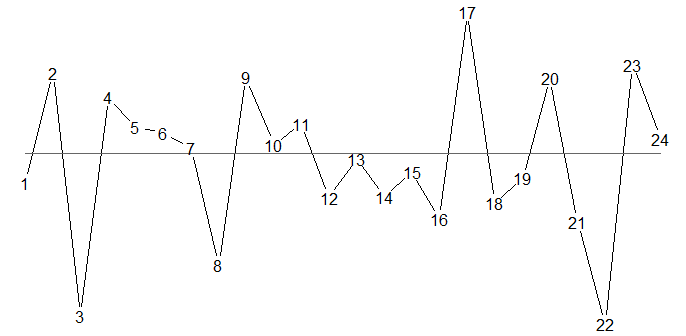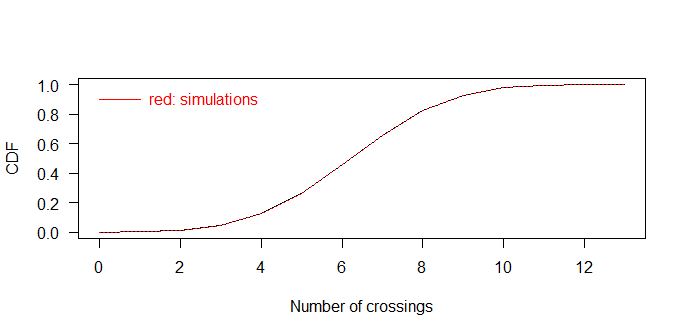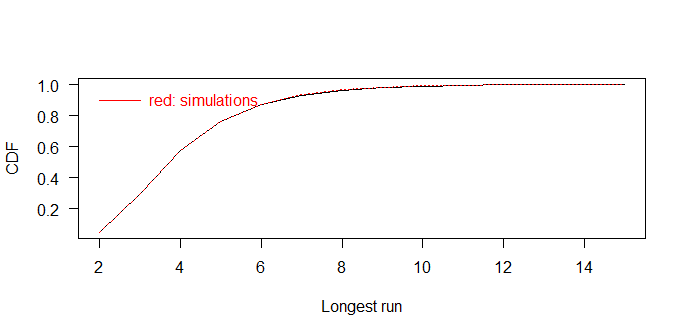# crossrun: An R Package for the Joint Distribution of Number of Crossings and Longest Run in Independent Bernoulli Observations

Centre for Child and Adolescent Mental Health, Eastern and Southern Norway; Norwegian Centre of Violence and Traumatic Stress Studies
tore.wentzellarsen@gmail.com
Rigshospitalet, University of Copenhagen, Denmark
jacob@anhoej.net

## Introduction

The setting is defined by a number, n, of independent observations from a Bernoulli distribution with equal success probability. In statistical process control, our main intended application, this may be the useful observations in a run chart recording values above and below a pre-specified centre line (usually the median obtained from historical data) disregarding any observations equal to the centre line (Anhøj (2015)).

The focus of crossrun is the number of crossings, C, and the length of the longest run in the sequence, L. A run is a sequence of successes or failures, delimited by a different observation or the start or end of the entire sequence. A crossing is two adjacent different observations.

Figure 1 illustrates runs and crossings in a run chart with 24 observations. Observations above and below the median represent successes and failures respectively:Figure 1

The longest run consists of observations 12-16 below the median. Thus, the length of the longest run is L = 5 in this case. The number of crossings of the median is C = 11.

C and L are inversely related. All things being equal, when one goes up, the other goes down. crossrun computes the joint distribution of C and L.

L and C are integers. L may be any integer between 1, if all subsequent observations are different, and n, if all observations are equal. C may be any integer between 0, if all observations are equal, and n - 1 if all subsequent observations are different. Not all combinations of C and L are possible as shown in the following example for n = 15 and success probability = 0.5.

Table 1
L = 1 2 3 4 5 6 7 8 9 10 11 12 13 14 15
C = 0 0 0 0 0 0 0 0 0 0 0 0 0 0 0 1
1 0 0 0 0 0 0 0 2 2 2 2 2 2 2 0
2 0 0 0 0 1 9 18 18 15 12 9 6 3 0 0
3 0 0 0 4 48 88 84 60 40 24 12 4 0 0 0
4 0 0 1 100 280 270 175 100 50 20 5 0 0 0 0
5 0 0 50 530 666 420 210 90 30 6 0 0 0 0 0
6 0 0 357 1197 861 392 147 42 7 0 0 0 0 0 0
7 0 8 1008 1456 672 224 56 8 0 0 0 0 0 0 0
8 0 84 1470 1044 324 72 9 0 0 0 0 0 0 0 0
9 0 252 1200 450 90 10 0 0 0 0 0 0 0 0 0
10 0 330 550 110 11 0 0 0 0 0 0 0 0 0 0
11 0 220 132 12 0 0 0 0 0 0 0 0 0 0 0
12 0 78 13 0 0 0 0 0 0 0 0 0 0 0 0
13 0 14 0 0 0 0 0 0 0 0 0 0 0 0 0
14 1 0 0 0 0 0 0 0 0 0 0 0 0 0 0

As will be described and justified in more detail later, the table above does not give the probabilities themselves, but the probabilities multiplied by $$2^{n-1}$$, that is 16384 for n = 15. For instance $$P (C=6, L=5)$$ = 861 / 16384 = 0.053. The highest joint probability is $$P (C=8, L=3)$$ = 1470 / 16384 = 0.090. Thus, in a run chart with 15 observations not on the median, the most likely longest run and number of crossings is 3 and 8 respectively.

As seen in the table, the non-zero probabilities are confined to a sloped region that is rather narrow but sufficiently wide that the two variables together are more informative than each of them in isolation. These are general phenomena.

The procedure for computing the joint distribution of C and L is iterative, which means that the joint distribution for a sequence of length n cannot be computed before the joint distributions for all shorter sequences have been computed. At the moment, the computations have been validated for n up to 100, and success probabilities 0.5 to 0.9 in steps of 0.1.

## The main function: crossrunbin

The function crossrunbin has two main arguments, nmax that is the maximum sequence length and prob that is the success probability. Other arguments include a multiplier described later and a precision parameter, these should normally be left at their default values. See ?crossrunbin for details.

The joint probabilities are stored in a list of 6 lists of which pt is sufficient for normal use. The others are mainly included for code checking. pt gives an n by n matrix for each sequence length n. For instance

crb40.6 <- crossrunbin(nmax = 40, prob = 0.6)$pt computes the joint probabilities for all sequence lengths $$n \leq 40$$ when the success probability is 0.6. For simplicity, the command above only returns the joint probabilities pt. When the computation is finished, the joint distribution for say n = 15 is crb40.6[] Actually the resulting joint distribution is not quite a matrix, it is a two-dimensional mpfr array (Fousse et, al, 2007, Mächler 2018). Two-dimensional mpfr arrays are almost the same as matrices, but with appreciably higher precision. Since the computation procedure is iterative, high precision during calculation is vital, but the resulting joint distributions may subsequently be transformed into ordinary matrices by the Rmpfr function asNumeric for easier presentation. To limit the package size, only the joint distributions for n = 15, 60, 100 and success probabilities 0.5 (the symmetric case) and 0.6 have been included in this package, as ordinary matrices. ## The “times” representation of the joint distributions As mentioned, the joint distributions are actually not computed as probabilities, but as probabilities multiplied by a multiplier whose default value is $$2^{n-1}$$. Optionally another multiplier $$m^{n-1}$$ could be used where $$m$$ is an argument (mult) to the function crossrunbin, but the default value should normally be used. This representation is shown in Table 1 for n = 15 and p = 0.5. One may note that in Table 1 all probabilities are represented by integers in the “times” representation. This is a general phenomenon in the symmetric case, but not for success probabilities different from 0.5. In the symmetric case (Anhøj and Vingaard Olesen (2014)) the number, C, of crossings has a binomial distribution with number of observations n - 1 and probability 0.5, and their marginal probabilities are just the binomial coefficients divided by $$2^{n-1}$$. Indeed, the row sums in the times representation are the binomial coefficients in the symmetric case. This will be explicated later in the case of n = 15. When the success probability is not 0.5, the joint distribution is no longer represented by integers even in the times representation. This is illustrated below for n = 15 and success probability 0.6. Table 2 L = 1 2 3 4 5 6 7 8 9 10 11 12 13 14 15 C = 0 0.0 0.0 0.0 0.0 0.0 0.0 0.0 0.0 0.0 0.0 0.0 0.0 0.0 0.0 7.7 1 0.0 0.0 0.0 0.0 0.0 0.0 0.0 1.5 1.8 2.3 3.2 4.7 6.9 10.3 0.0 2 0.0 0.0 0.0 0.0 1.1 11.4 29.3 33.7 31.8 29.7 26.8 21.9 13.8 0.0 0.0 3 0.0 0.0 0.0 3.0 41.7 91.7 105.9 87.8 70.3 51.9 32.6 13.9 0.0 0.0 0.0 4 0.0 0.0 0.9 99.2 318.3 354.3 260.6 172.1 101.9 49.2 15.1 0.0 0.0 0.0 0.0 5 0.0 0.0 39.3 468.8 670.6 483.1 278.4 141.5 57.1 14.1 0.0 0.0 0.0 0.0 0.0 6 0.0 0.0 319.2 1180.3 948.8 485.7 210.1 70.9 14.2 0.0 0.0 0.0 0.0 0.0 0.0 7 0.0 6.0 836.1 1324.0 679.9 257.7 75.4 12.9 0.0 0.0 0.0 0.0 0.0 0.0 0.0 8 0.0 68.5 1289.2 994.7 341.5 86.5 12.6 0.0 0.0 0.0 0.0 0.0 0.0 0.0 0.0 9 0.0 196.4 991.5 402.9 89.8 11.5 0.0 0.0 0.0 0.0 0.0 0.0 0.0 0.0 0.0 10 0.0 267.1 466.8 100.8 11.3 0.0 0.0 0.0 0.0 0.0 0.0 0.0 0.0 0.0 0.0 11 0.0 170.3 106.7 10.5 0.0 0.0 0.0 0.0 0.0 0.0 0.0 0.0 0.0 0.0 0.0 12 0.0 61.2 10.6 0.0 0.0 0.0 0.0 0.0 0.0 0.0 0.0 0.0 0.0 0.0 0.0 13 0.0 10.5 0.0 0.0 0.0 0.0 0.0 0.0 0.0 0.0 0.0 0.0 0.0 0.0 0.0 14 0.8 0.0 0.0 0.0 0.0 0.0 0.0 0.0 0.0 0.0 0.0 0.0 0.0 0.0 0.0 In Table 2 the results are shown with one decimal. The cells different from 0 are the same as in the symmetric case, but the distribution centre has been shifted in the direction of longer runs and fewer crossings. The times representation may be advantageous for presentation because very small numbers are avoided. However, the main reason for using this representation is to enhance precision in the iterative computation procedure. ## The symmetric case: crossrunsymm In the symmetric case the joint probabilities are, as illustrated in Table 1, stored as integers in the times representation. A separate function crossrunsymm is available in this case. The arguments, except the success probability, are the same as in the more general function crossrunbin, but the inner workings are somewhat simpler. ## Generalisation In the case of variable success probability, a similar procedure is available and implemented in the function crossrunchange. In this procedure all arguments are as in crossrunbin, except that the success probability is replaced by a vector of length n with success probabilities for each of the n time points. ## Limitations The main limitation of this method is that the procedure does only apply when the median is pre-specified, not when it is determined by the time series itself, which, in practice, is often the case when using run charts for real time process monitoring. Also, the procedure cannot be generalized to autocorrelated time sequences. ## Conclusions The crossrun package is, to our knowledge the first software package that allows for the computation of joint probabilities of longest run and number of crossings in time series data. This is an important step forward, as previous work on the subject have only dealt with these parameters as independent entities. This work may form the basis of better tests for non-random variation in time series data than are currently available. ## Appendix: Checking procedures Procedures for checking the joint distributions are available in crossrun. First, the function exactbin computes the joint distribution for $$n \leq 6$$ independently of the iterative procedure, by formulas based on “brute force” enumeration that is practically feasible for these short sequences. An example of use of exactbin for checking of results of the exact procedure is as follows, for n=5 and success probability 0.6 (multiplied by $$2^4=16$$ to conform with the times representation): library(crossrun) library(Rmpfr) exact1 <- asNumeric((2^4) * exactbin(n = 5, p = 0.6)) iter1 <- asNumeric(crossrunbin(nmax = 5, prob = 0.6)$pt[])
compare1 <- cbind(exact1,iter1)

compare1
#>        1      2      3      4     5      1      2      3      4     5
#> 0 0.0000 0.0000 0.0000 0.0000 1.408 0.0000 0.0000 0.0000 0.0000 1.408
#> 1 0.0000 0.0000 1.8432 2.1504 0.000 0.0000 0.0000 1.8432 2.1504 0.000
#> 2 0.0000 2.9184 3.0720 0.0000 0.000 0.0000 2.9184 3.0720 0.0000 0.000
#> 3 0.0000 3.6864 0.0000 0.0000 0.000 0.0000 3.6864 0.0000 0.0000 0.000
#> 4 0.9216 0.0000 0.0000 0.0000 0.000 0.9216 0.0000 0.0000 0.0000 0.000

Here the 5 leftmost columns come from exact calculations while the 5 rightmost columns come from the iterative procedure. The maximum absolute difference is computed as 0 in this case. Generally some tiny differences may occur.

The iterative computations may also be checked for appreciably higher n. As commented above the row sums in the symmetric case are just the binomial coefficients. The following code checks this fact for n=15.

library(crossrun)
library(Rmpfr)

bincoeff14           <- Rmpfr::chooseMpfr.all(14) # binomial coefficients, n - 1 = 14
bincoeff14iter       <- cumsumm(j15s)[-1, 15]     # row sums, n - 1 = 14
compare15            <- rbind(asNumeric(bincoeff14), bincoeff14iter)
row.names(compare15) <- c("exact","iter")

compare15
#>        1  2   3    4    5    6    7    8    9   10  11 12 13 14
#> exact 14 91 364 1001 2002 3003 3432 3003 2002 1001 364 91 14  1
#> iter  14 91 364 1001 2002 3003 3432 3003 2002 1001 364 91 14  1
max(abs(bincoeff14 - bincoeff14iter))
#> 1 'mpfr' number of precision  53   bits
#>  0

This check has been repeated for $$n \leq 100$$ with full mpfr precision without finding any discrepancies.

The results of the iterative procedure may also be checked by results of simulations. The crossrun function simclbin performs simulations for the number of crossings and the longest run for chosen values of the success probability. Again, computations for substantially longer sequences (n appreciably higher than 15) should use full mpfr precision for the joint distribution. The following code shows the procedure for n = 15 and 10000 simulations and compares the mean of $$C \cdot L$$ in the simulations with the corresponding mean from the joint distribution p6.15 with success probability 0.6:

library(crossrun)
set.seed(83938487)
sim15 <- simclbin(nser = 15, nsim = 10000)
(matrix(0:14, nrow = 1) %*% p6.15 %*% matrix(1:15, ncol = 1)) / 2^14
#>          [,1]
#> [1,] 28.30112
mean(sim15$nc0.6 * sim15$lr0.6)
#>  28.3145

Here, $$C \cdot L$$ is just used as an example of a fairly demanding function of the number C of crossing and the longest run L. Again, computations for substantially longer sequences (n appreciably higher than 15) should use full mpfr precision for the joint distribution. Simpler statistics include means and standard deviations of C and L separately. The following shows a graphical comparison of the cumulative distribution functions of C and L based on the joint distribution and the simulations.